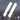# HTTP 之重定向

June 06, 2020

HTTP

## 前言

Oh No，这个回答简直烂大街，何不以另一种方式来解答。

• 临时重定向（Temporary Redirect），每次重定向不影响下一次访问
• 永久重定向（Permanent Redirect），在第一次重定向后，除非用户主动清除缓存，否则后续访问都命中第一次重定向的结果，即使服务端代码发生更改

## “暗藏 bug”的重定向：301、302

``````├── A.html
├── B.html
└── app.js``````
``````// app.js
const http = require("http");
const fs = require("fs");

const server = http.createServer((req, res) => {
if (req.url === "/a") {
res.statusCode = 302;
res.end();
}
if (req.url === "/b") {
}
});

server.listen(3000, () => {
console.log("server start!");
});``````
``````<!-- A.html -->
<!DOCTYPE html>
<html lang="en">
<meta charset="UTF-8" />
<meta name="viewport" content="width=device-width, initial-scale=1.0" />
<title>Document</title>
<body>
<h2>I'm page A</h2>
</body>
</html>``````
``````<!-- B.html -->
<!DOCTYPE html>
<html lang="en">
<meta charset="UTF-8" />
<meta name="viewport" content="width=device-width, initial-scale=1.0" />
<title>Document</title>
<body>
<h2>I'm page B that redirects from page A</h2>
</body>
</html>````````````const server = http.createServer((req, res) => {
if (req.url === "/a") {
// res.statusCode = 302;
// res.end();
}
if (req.url === "/b") {
}
});``````

``````const server = http.createServer((req, res) => {
if (req.url === "/a") {
res.statusCode = 301;
res.end();
}
if (req.url === "/b") {
}
});````````````const server = http.createServer((req, res) => {
if (req.url === "/a") {
// res.statusCode = 301;
// res.end();
}
if (req.url === "/b") {
}
});````````````<!DOCTYPE html>
<html lang="en">
<meta charset="UTF-8" />
<meta name="viewport" content="width=device-width, initial-scale=1.0" />
<title>Document</title>
<body>
<button>send POST request</button>
<script>
const btn = document.querySelector("button");
btn.onclick = () => {
fetch("http://localhost:3000/a", { method: "post" })
.then(res => res.text())
.then(data => console.log(data));
};
</script>
</body>
</html>``````

``````const server = http.createServer((req, res) => {
if (req.url === "/") {
}
if (req.url === "/a" && req.method === "POST") {
res.statusCode = 302;
res.end();
}
if (req.url === "/b" && req.method === "POST") {
res.end("data redirects from b");
}
if (req.url === "/b") {
res.end("what's wrong");
}
});``````## “实至名归”的重定向：307、308

• 308（Permanent Redirect）替代 301
• 307（Temporary Redirect）替代 302

``````if (req.url === "/a" && req.method === "POST") {
res.statusCode = 307;
res.end();
}``````## 总结

Permanent Temporary

RFC 7538Written by B2D1（包邦东）